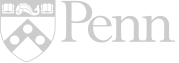## Search ResultsView by: Year | Seminar

Unit TitleAuthor

### Creating a Survey and Analyzing and Displaying the Data Results from a Survey

Lance M. Levy
Keywords: Algebra 1, Math, Probability / Statistics, Probability and Statistics, statistical distributions, statistical questions, Statistics

### Know Your Position – How?

Ann Cherian
Keywords: binomial theory, binomial probability, decision making, statistical distributions, Pascal's Triangle, Probability and Statistics, measures, Statistics, tools, normal distribution